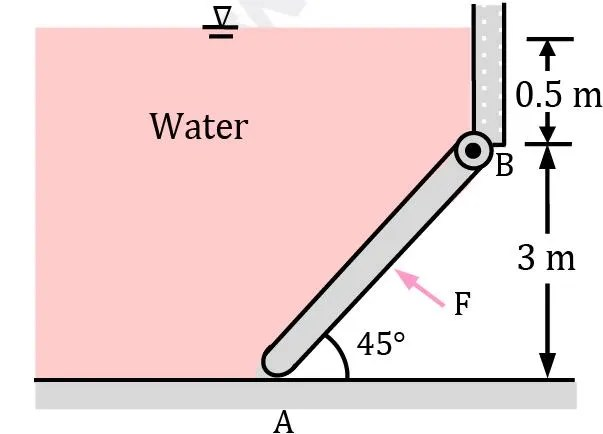# The 200-kg, 5-m-wide rectangular gate shown in figure is hinged at B and leans against the floor at A making an angle of 45° with the horizontal. The gate is to be opened from its lower edge by applying a normal force at its center. Determine the minimum force F required to open the water gate.

Question-AnswerCategory: Fluid MechanicsThe 200-kg, 5-m-wide rectangular gate shown in figure is hinged at B and leans against the floor at A making an angle of 45° with the horizontal. The gate is to be opened from its lower edge by applying a normal force at its center. Determine the minimum force F required to open the water gate.

The 200-kg, 5-m-wide rectangular gate shown in figure is hinged at B and leans against the floor at A making an angle of 45° with the horizontal. The gate is to be opened from its lower edge by applying a normal force at its center. Determine the minimum force F required to open the water gate.a) 420

b) 520

c) 620

d) 720For the surface the gate
hc = 2 m
∴ hp = hc + IG / Ahc sin2θ
hp = 2 + 5×(3√2) 3 / 12 / 5 × 3√2 × 2 × 1 / 2
hp = 2 + 9 × 2 / 12 × 2×1 / 2
hp = 2.375 mAlso Fp = ρgAhc
Fp = 1000 × 9.81 × (5 × 3√2) × 2
Fp = 416.2 kN
Fp × 1.875√2 + w × 1.5 = F × 1.5√2
F = 521 kN